Open in App
Not now

# How to Iterate through each element in N-Dimensional matrix in MATLAB?

• Difficulty Level : Basic
• Last Updated : 20 Oct, 2021

In MATLAB, a matrix is considered a two-dimensional array of numbers. Other programming languages work with numbers but in MATLAB, every number is a matrix or array. To create a matrix in MATLAB, numbers are entered in each row by adding a comma or space and the ending of each row is marked by a semicolon.

Suppose, the following code created a matrix of 2-by-2:

Example:

## Matlab

 `% MATLAB Code for 2x2 matrix  ` `M = [1 2 ; 3 4]`

Output: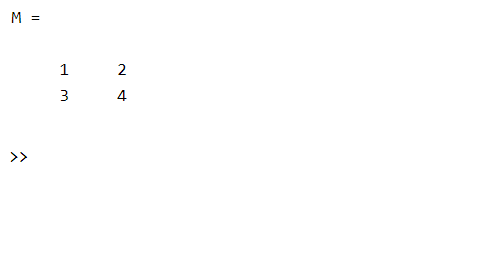## Method 1

In this method, we iterate a matrix when you need to keep track of the index at which you are currently at present.

Example:

## Matlab

 `% MATLAB Code for iteration ` `% Create a matrix of 3-by-4 dimension ` `M = [2 3 4 5; ` `    ``6 7 8 9 ; ` `    ``0 1 6 8]; ` ` `  `% Get rows and columns of matrix using ` `% size() function ` `[rows,column]=size(M); ` ` `  `% Iterate through each row and display  ` `% each column element of that row ` `for` `i=1:rows ` `for` `j=1:column ` `x=M(i,j); ` `fprintf( ``'   %d    '``,x); ` `end` `    `  `% Add a line break after one row is displayed ` `fprintf(``'\n'``); ` `end`

Output: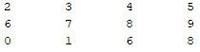output matrix

## Method 2

The above method to iterate a matrix is used when you need to keep track of the index at which you are currently at present. There is another way to iterate a matrix using a semicolon. In the matrix, as discussed above, each element can be accessed by specifying its position in the matrix. If you want to iterate through some specific elements use range based indexing as illustrates below:

Example 1:

## Matlab

 `% MATLAB Code for iteration using semicolon ` `% Create a matrix of 3-by-4 dimension ` `M=[2 3 4 5; 6 7 8 9 ; 0 1 6 8]; ` ` `  `%Most often in matrix we use vectors subscripts  ` `% to access elements in specific range. For example ` `% to access rows 2nd and 3rd, 1st and 2nd column use indexing as: ` `M(2:3,1:2) ` ` `  `%2:3 specifies that extract elements of 2nd and 3rd row  ` `%1:2 for column index tells to extract 1st and 2nd column elements.`

Output: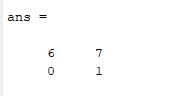OUTPUT

Similarly, the whole matrix can be iterated by using range based indexing as:

Example 2:

## Matlab

 `% MATLAB Code for the whole matrix iteration ` `% by using range based indexing  ` `% Create a matrix of 3-by-4 dimension ` `M=[2 3 4 5; 6 7 8 9 ; 0 1 6 8]; ` ` `  `%similarly if you want to iterate through whole matrix, just specify its ` `%rows and columns ` `M(1:3,1:4)``%iterate through rows 1-3 and column 1-4.`

Output: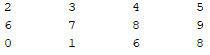OUTPUT

Extending the above idea, the whole matrix can be iterated without explicitly defining range vectors as shown below:

Example 3:

## Matlab

 `% MATLAB Code for whole matrix can be iterated  ` `% without explicitly defining range vectors ` `%Create a matrix of 3-by-4 dimension ` `M=[2 3 4 5; 6 7 8 9 ; 0 1 6 8]; ` ` `  `%Leave rows and column place as empty and  ` `% MATLAB takes it as 'all'  ` `M(:,:) ` ` `  `%whole matrix iterated.`

Output:OUTPUT

## Method 3

In MATLAB there is a function numel that can give the number of elements in a matrix. Using it iterate through the matrix and display each element of the matrix as shown below:

Example:

## Matlab

 `% MATLAB Code for iteration using numel()  ` `% Create a matrix of 3-by-4 dimension ` `M=[2 3 4 5; 6 7 8 9 ; 0 1 6 8]; ` ` `  `% create output vector for storing outputs ` `output=[]; ` ` `  `% get number of elements in matrix M ` `n=numel(M); ` ` `  `% loop through all elements and store values in output array. ` `for` `i=1:n ` `output(i)=M(i); ` `end` ` `  `% prints output array. ` `output`

Output: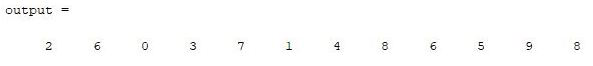OUTPUT

Here the output is different from above because elements of a matrix are accessed column-wise. As the loop goes from 1 to a number of elements in the matrix, every element is accessed according to its index. The table shows the linear indexing of each element in the MATLAB matrix.

My Personal Notes arrow_drop_up
Related Articles